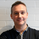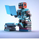1843 obejrzeń
1843
The author of this indicator is Veronique Valcu. The z-score (z) for a data
item x measures the distance (in standard deviations StdDev) and direction
of the item from its mean (U):
z = (x-StdDev) / U
A value of zero indicates that the data item x is equal to the mean U, while
positive or negative values show that the data item is above (x>U) or below
(x Values of +2 and -2 show that the data item is two standard deviations
above or below the chosen mean, respectively, and over 95.5% of all data
items are contained within these two horizontal references (see Figure 1).
We substitute x with the closing price C, the mean U with simple moving
average ( SMA ) of n periods (n), and StdDev with the standard deviation of
closing prices for n periods, the above formula becomes:
Z_score = (C - SMA (n)) / StdDev(C,n)
The z-score indicator is not new, but its use can be seen as a supplement to
Bollinger bands . It offers a simple way to assess the position of the price
vis-a-vis its resistance and support levels expressed by the Bollinger Bands .
In addition, crossings of z-score averages may signal the start or the end of
a tradable trend. Traders may take a step further and look for stronger signals
by identifying common crossing points of z-score, its average, and average of average.
```////////////////////////////////////////////////////////////
//  Copyright by HPotter v1.0 07/07/2014
// The author of this indicator is Veronique Valcu. The z-score (z) for a data
// item x measures the distance (in standard deviations StdDev) and direction
// of the item from its mean (U):
//     z = (x-StdDev) / U
// A value of zero indicates that the data item x is equal to the mean U, while
// positive or negative values show that the data item is above (x>U) or below
// (x Values of +2 and -2 show that the data item is two standard deviations
// above or below the chosen mean, respectively, and over 95.5% of all data
// items are contained within these two horizontal references (see Figure 1).
// We substitute x with the closing price C, the mean U with simple moving
// average (SMA) of n periods (n), and StdDev with the standard deviation of
// closing prices for n periods, the above formula becomes:
//     Z_score = (C - SMA(n)) / StdDev(C,n)
// The z-score indicator is not new, but its use can be seen as a supplement to
// Bollinger bands. It offers a simple way to assess the position of the price
// vis-a-vis its resistance and support levels expressed by the Bollinger Bands.
// In addition, crossings of z-score averages may signal the start or the end of
// a tradable trend. Traders may take a step further and look for stronger signals
// by identifying common crossing points of z-score, its average, and average of average.
////////////////////////////////////////////////////////////
study(title="Z-Score", shorttitle="Z-Score")
Period = input(20, minval=1)
hline(0, color=purple, linestyle=line)
xStdDev = stdev(close, Period)
xMA = sma(close, Period)
nRes = (close - xMA) / xStdDev
plot(nRes, color=blue, title="Z-Score")```
DONATE/TIP

BTC: 3FKWwtaYrf5NHZzaCi1fyAfQj7XSgtyCqe

## KomentarzeThanks @HPotter !

Can you clarify this: "identify common crossing points of z-score" ?

Any example scripts of this anywhere? :)
OdpowiedźThanks. Very useful indicator! Could you possibly Normalize the data into price bars in a lower panel?

OdpowiedźGreat stuff Porter...this will be very useful for statistical arbitrage. Thanks for sharing
Odpowiedź